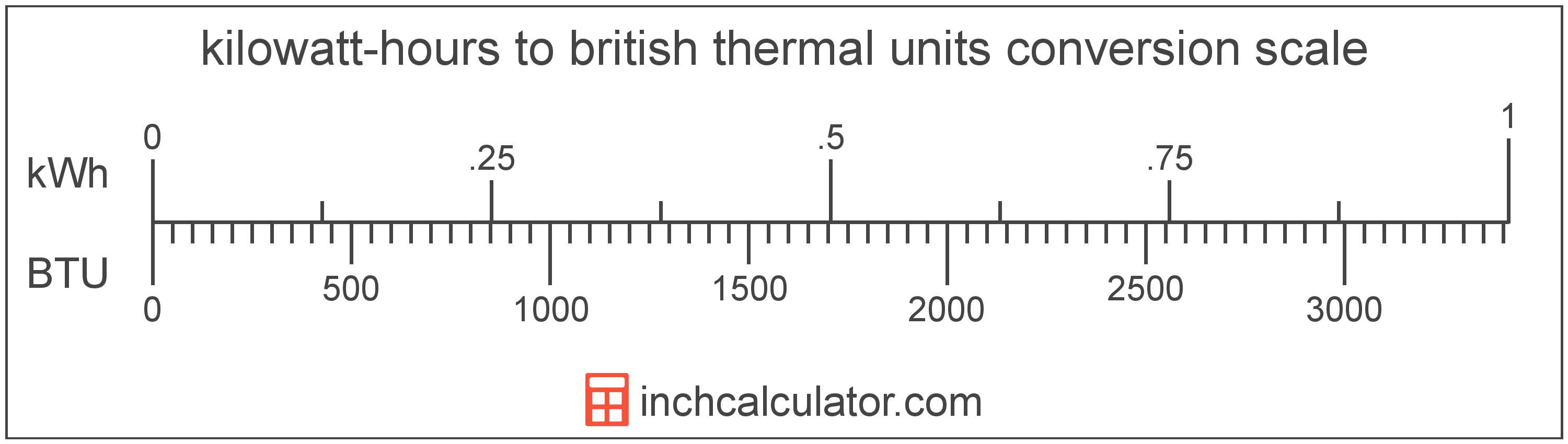# British Thermal Units to Kilowatt-Hours Converter

Enter the energy in british thermal units below to get the value converted to kilowatt-hours.

Results in Kilowatt-Hours:1 BTU = 0.000293 kWh

Do you want to convert kilowatt-hours to british thermal units?

## How to Convert British Thermal Units to Kilowatt-Hours

To convert a measurement in british thermal units to a measurement in kilowatt-hours, multiply the energy by the following conversion ratio: 0.000293 kilowatt-hours/british thermal unit.

Since one british thermal unit is equal to 0.000293 kilowatt-hours, you can use this simple formula to convert:

kilowatt-hours = british thermal units × 0.000293

The energy in kilowatt-hours is equal to the energy in british thermal units multiplied by 0.000293.

For example, here's how to convert 5,000 british thermal units to kilowatt-hours using the formula above.
kilowatt-hours = (5,000 BTU × 0.000293) = 1.465355 kWh## What is a British Thermal Unit?

British thermal units are a measure of heat energy. Specifically, one british thermal unit is equal to the amount of heat energy required to increase the temperature of one pound of water one degree Fahrenheit. They are often used as a way to rate the energy heat producing appliances such furnaces, and to price heating fuels such as natural gas.

The british thermal unit is a US customary unit of energy. British thermal units can be abbreviated as BTU; for example, 1 british thermal unit can be written as 1 BTU.

## What is a Kilowatt-Hour?

A kilowatt-hour is a measure of electrical energy equal to one kilowatt, or 1,000 watts, of power over a one hour period. Kilowatt-hours are a measure of electrical work performed over a period of time, and are often used as a way of measuring energy usage by electric companies.

Kilowatt-hours are usually abbreviated as kWh, although the formally adopted expression is kW·h. The abbreviation kW h is also sometimes used. For example, 1 kilowatt-hour can be written as 1 kWh, 1 kW·h, or 1 kW h.

In formal expressions, the centered dot (·) or space is used to separate units used to indicate multiplication in an expression and to avoid conflicting prefixes being misinterpreted as a unit symbol.

## British Thermal Unit to Kilowatt-Hour Conversion Table

Table showing various british thermal unit measurements converted to kilowatt-hours.
British Thermal Units Kilowatt-hours
1 BTU 0.000293 kWh
2 BTU 0.000586 kWh
3 BTU 0.000879 kWh
4 BTU 0.001172 kWh
5 BTU 0.001465 kWh
6 BTU 0.001758 kWh
7 BTU 0.002051 kWh
8 BTU 0.002345 kWh
9 BTU 0.002638 kWh
10 BTU 0.002931 kWh
20 BTU 0.005861 kWh
30 BTU 0.008792 kWh
40 BTU 0.011723 kWh
50 BTU 0.014654 kWh
60 BTU 0.017584 kWh
70 BTU 0.020515 kWh
80 BTU 0.023446 kWh
90 BTU 0.026376 kWh
100 BTU 0.029307 kWh
200 BTU 0.058614 kWh
300 BTU 0.087921 kWh
400 BTU 0.117228 kWh
500 BTU 0.146536 kWh
600 BTU 0.175843 kWh
700 BTU 0.20515 kWh
800 BTU 0.234457 kWh
900 BTU 0.263764 kWh
1,000 BTU 0.293071 kWh
2,000 BTU 0.586142 kWh
3,000 BTU 0.879213 kWh
4,000 BTU 1.1723 kWh
5,000 BTU 1.4654 kWh
6,000 BTU 1.7584 kWh
7,000 BTU 2.0515 kWh
8,000 BTU 2.3446 kWh
9,000 BTU 2.6376 kWh
10,000 BTU 2.9307 kWh

## References

1. Bureau International des Poids et Mesures, The International System of Units (SI), 9th edition, 2019, https://www.bipm.org/documents/20126/41483022/SI-Brochure-9-EN.pdf